1. Bibliography

1.1. In English

• Pagina (oficială) de pornire used for documentation; the main topics are:

• The Python Tutorial -- a perfect starting point for those that want to start programming in Python: it covers the basis of Python programming;

• Using Python -- it describes the installation and usage of the Python interpreter in the context of main operating systems;

• The Python Language Reference -- a good cover material for the Python language (syntax and basic entities);

• The Python Standard Library -- the cover material for all standard Python libraries (practically all that there is to know about Python);

2. Introduction

What is python? ::

• First of all an programming language, but also an execution environment (run-time environment);

What does the term language mean in the context of computers? ::

• A method in which the user (not necessarily a programmer) interacts with an application.

In general we are thinking at a text with a well defined format that is understood by the application.

What does programming imply?

• It referrers to the possibility of prescribing a sequence of actions that an application must undertake.

In general the programming has two flavors:

• temporary (after the application restarts the sequence of actions is lost);

• or permanent;

Programming language?

• The way in which the actions are prescribed for a certain application (or for the computer itself).

The text that results is usually called source code, or simpler said program.

Program execution?

• The application uses the source code (described according to the rules in the programming language) and undertakes the actions prescribed.

What is an shell?

• The way in which some applications obtain the source code can differ. But the majority falls in one of the two use cases:
• either the source code is provided as a computer file;

• either the interaction with the user takes the form of a dialogue.

The applications that fall in the last category are generically called shells. (Python fits both categories.)

3. Dialoguing with Python

3.1. Starting the shell

Both under Windows and under Linux we could use IDLE (An Integrated DeveLopment Environment for Python). This is accessible from the menus of both operating systems.

(Furthermore under Linux we could use also the built-in Python shell python, or ipython.)

Once started, Python informs us about the current version, and displays a prompt (a sequence of characters that indicates to the user that the application waits for commands):

   1 #Python 2.6 ...
2 >>>


3.2. "Pocket calculator"

Although we have in our hands a very complex and powerful application, we can "downgrade" it and use it as a simple pocket calculator.

But before continuing we must be aware of a few issues:

• in the case we copy/paste the examples that follow in this text, we must remember that the user does not have to input the characters >>>, but only what follows after it.

• in general every shell while dialoguing with the user behaves in the following manner:

1. the shell displays a prompt (in the case of Python this is >>>);

2. the user inputs the text (conforming to the rules of the language understood by the application), followed by the Enter key;

3. the shell tries to parse the code (accordingly with the language), and in the case it understands what it has to do, it undertakes the action;

4. and as a consequence of the execution of the requested actions, the shell displays the outcome of the operation; (this is not always the case;)

Simple operations:

•    1 >>> 1 + 2
2 3
3 >>> 2 * 3 + 4
4 10
5 >>> 2 ** 3
6 8


Prioritized operations:

•    1 >>> (1 + 2) * 3
2 9


Operations with complex numbers:

•    1 >>> 3j ** 2
2 (-9+0j)
3 >>> (2 + 3j) ** 2
4 (-5+12j)


3.3. Numeric data types

• integers: 1, 259, -874;

• reals:
• in decimal format: 0.0, -895.059;

• in scientific format: 1.7e+5, -59.7e-80;

• complex:
• with only an imaginary part: 7j, -9.8884j, 5.9e200j);

• with only a real part: (3+0j), (-2.75+0j;

• both real and imaginary parts: (15+20j);

3.4. Arithmetic operators

• +, - -- addition, subtraction;

• * -- multiplication;

• / -- division, but:

• if we divide two integer numbers then the result represents only the integer part: 3 / 2 => 1, 1 / 2 => 0;

• in any other case the division is an exact one: 3 / 2.0 => 0.5, 1.0 / 2 => 0.5;

• % -- the reminder of the division, but:

• we could use it even for real numbers: 0.7 % 0.3 => 0.09999...

• ** -- power raising;

3.5. Arithmetic functions

• abs -- absolute value:

•    1 >>> abs(5)
2 5
3 >>> abs(-1)
4 1
5 >>> abs(-9.72)
6 9.72
7 >>> abs(1+3j)
8 3.162

• min, max:

•    1 >>> min(5,9)
2 5
3 >>> max(5,9)
4 9

• round -- rounding:

•    1 >>> round(5.6)
2 6
3 >>> round(5.5)
4 6
5 >>> round(5.1)
6 5
7 >>> round(-1.5)
8 2

• int -- real to integer conversion (by truncation):

•    1 >>> int(5.5)
2 5
3 >>> int(-1.5)
4 -1


3.6. Variables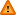In contrast to other programming languages, in Python there is no need of declaring variables, instead these are automatically defined in the moment of assignment.

   1 >>> pi = 3.14
3 >>> perimeter = 2 * pi * radius
4 >>> perimeter
5 43.96
6 >>> surface = pi * radius ** 2
7 >>> surface
8 153.86


3.7. Other mathematical functions

There are some useful functions that are held in the mathematical module (see the documentation for a complete list).

Importing the functions:

•    1 # if we need the sqrt function
2 from math import sqrt
3
4 # or if we need the trigonometric functions
5 from math import sin, cos, tan


Using the functions:

•    1 r = sqrt (90)


4. Programming in Python

4.1. Source code editing

For IDLE: File -> New Window (Control-N) or File -> Open... (Control-O).

For Linux in the command line: nano <path-of-file>.

(The extension for Python files is .py.)

4.2. Source code execution

For IDLE: Run -> Run Module (F5.

For Linux in the command line: python <path-of-file>.

Generic syntax:

•    1 z = x + y # afther the # character follows a comment until the end of the line
2 # w = x * y -- this entire line is a comment, and the w variable is not initialized


5. Outputting to the console

Generic syntax:

•    1 print
2 print value_1, value_2, ..., value_n


Examples:

• >>> x = 1
>>> print "a =", x
a = 1
>>> print "a =", "x"
a = x
>>> print

>>> print 5
5

6. Control structures

6.1. Conditional structureAlso in contrast to other programming languages, in Python correct (consistent) indentation (the spaces at the beginning of lines) is obligatory. It is recommended to use 4 spaces for each indentation level, or a single tab character. However it is not recommended to mix the two styles.

Generic syntax:

•    1 if condition :
2         operation
3         ...
4 else :
5         operation
6         ...


The syntax without the else clause:

•    1 if condition :
2         operation
3         ...


The syntax for multiple conditions:

•    1 if condition_1 :
2         operation
3         ...
4 elif condition_2 :
5         operation
6         ...
7 ...
8 else :
9         operation
10         ...


6.1.1. Examples

Testing if a number is even:

•    1 x = 5
2 if x % 2 == 0 :
3         print "even"
4 else :
5         print "odd"


Simulating the absolute function abs:

•    1 x = -5
2 if x < 0 :
3         x = 0 - x
4 print x


6.2. Iterative structure

Iteration over an interval:

•    1 for i in range(min, max + 1) :
2         operation
3         ...


Iteration over an interval with a step:

•    1 for i in range(min, max + 1, step) :
2         operation
3         ...


Iteration over a list:

• for i in [element_1, element_2, ..., element_n] :
operation
...

6.2.1. Examples

The sum of numbers from 1 to 100 :

•    1 sum = 0
2 for i in range(1, 101) :
3         sum = sum + 1
4 print sum


Displaying the members of a list:

• lst = ["a", "b", "c"]
for e in lst :
print e

6.3. Repetitive structure

Generic syntax:

•    1 while condition :
2         operation
3         ...


6.3.1. Examples

The sum of the numbers from 1 to 100 :

•    1 sum = 0
2 i = 1
3 while i <= 100 :
4         sum = sum + i
5         i = i + 1
6 print sum


7. Lists

Creating lists:

•    1 # creating a list with some elements
2 l = [1, 2, 3]
3 print l
4
5 # creating a list of a certain size initialized with a certain value
6 l =  * 10
7 print l
8 # we should see: [0, 0, 0, 0, 0, 0, 0, 0, 0, 0]
9
10 print len(l)
11 # we should see: 10, the length of the list


Inputing lists:

•    1 l=input("l=") # and enter [1,2,3]
2 print l


Accessing and updating elements:

•    1 l = [1, 2, 3]
2 print l
3 print l
4 print l
5 l = 3
6 l = 2
7 print l
8 # we should see [1, 3, 2]


Adding an element at the end of a list:

•    1 l = []
2 l.append (1)
3 l.append (2)
4 print l
5 # we should see [1, 2]


Reversing the content of a list:

•    1 l = [1, 2, 3]
2 l.reverse ()
3 print l
4 # we should see [3, 2, 1]


8. Functions

Generic syntax:

•    1 def name (argument_1, argument_2, ...) :
2     operation
3     ...
4     return value


Example (returning a list of digits of a number):

•    1 def digits (n) :
2     dl = []
3     while n > 0 :
4         d = n % 10
5         n = n / 10
6         dl.append (d)
7     dl.reverse ()
8     return dl
9
10 print digits (1234)
11 # we should see [1, 2, 3, 4]


9. Problems

9.1. Coversions

1. Write a program that converts an angle from radians to degrees.
2. Write a program that converts an angle expressed in degrees in decimal form, into the following components: degrees (integer value), minutes, seconds, tens and hundreds of seconds.

9.2. Conditional structures

1. A point in 2D space is given by its coordinate x and y. Write a program that determines if the point is in the axis origin, or in the quadrants numbered from 1 to 4 (in reverse clock order), or if the point is on the border of the quadrants.
2. Considering a sphere expressed as its center coordinate and its radius, and given an arbitrary point in space, it should be determined its relative position to the sphere (i.e. inside, outside or on the surface).
3. Write a program that determines the ideal body weight of a person, given the waist, age an gender. The formula are:
•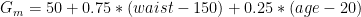•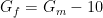4. Given the current date expressed as three integer numbers (year, month, and day), compute how many hours have elapsed since the beginning of the year, and how many hours are left until the end of the year. (We can consider that February has only 28 days.)
5. Given the current date expressed as three integer numbers (year, month, and day), and a persons birth-date expressed the same way, write a program that computes the age of the person, expressed as a number real number. (We consider that February has 28.25 days.)

9.3. Iterative structures

1. Write a program that determines the greatest common divisor of two natural non-negative numbers, using only subtraction.
2. Write a program that determines the greatest common divisor of two natural non-negative numbers, using Euclid's algorithm.
3. Write a program that determines the value of x raised to power n.
4. Write a program that determines if a number is prime.
5. Write a program that determines the sum of the digits for a number.
6. Write a program that determines the first n terms of the Fibonacci sequence.
7. Write a program that determines all the numbers smaller than n and are co-prime.
8. Considering the following natural number sequence: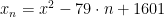, determine the percentage of the values for n in a given interval (from a to b), so that the value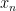is prime.

9. Write a program that determines all the perfect natural numbers that don't exceed a given value n.
10. Write a program that determines all the sequences of successive odd numbers, whose sum is n raised to the power 3. Ex.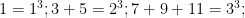.

11. Write a program that decomposes a given number n into its prime factors.
12. Write a program that determines the decomposition of a natural even number, greater than 2, as a sum of two prime numbers.

9.4. Economic problems

1. Considering the evolution of a bank deposit that is reconstituted each month, write a program that takes as inputs the initial deposited sum, the annual interest, and the number of months, and computes the final value of the deposit.
2. In the context of the previous problem, and by taking into consideration also the annual inflation (that we consider a constant given as an input), and applying this inflation at the end of each 12 months, compute the net value at the end of the period.